AtomicDensityMatrix`
If[StringContainsQ[Missing[KeyAbsent, Metadata][uri], StartOfString~~{A, B, C, D, E, F, G, H, I, J, K, L, M, N, O, P, Q, R, S, T, U, V, W, X, Y, Z}], Unevaluated[Sequence[XMLElement[span, {class -> main-title-top}, {Transmogrify`XMLTransformPrivate`context\$258671}], XMLElement[h1, {class -> main-title}, {Transmogrify`Recurse[]}], XML`RawXML[<div class="iconography" data-src="s= d= m= c= u= l= n= e="></div>], Transmogrify`XMLTransformPrivate`relatedInterpreterCell\$258671]], If[MemberQ[{Embedding Format, File, Front End Object, Interpreter, MathLink C Function, Method}, Transmogrify`GetParameter[entityType]], XMLElement[div, {class -> main-heading}, {XMLElement[h1, {class -> main-title}, {Transmogrify`Recurse[]}], XML`RawXML[<div class="iconography" data-src="s= d= m= c= u= l= n= e="></div>], Transmogrify`XMLTransformPrivate`relatedInterpreterCell\$258671}], Unevaluated[Sequence[XMLElement[h1, {class -> main-title}, {Transmogrify`Recurse[]}], XML`RawXML[<div class="iconography" data-src="s= d= m= c= u= l= n= e="></div>], Transmogrify`XMLTransformPrivate`relatedInterpreterCell\$258671]]]]

LevelDiagram[sys]

draws a level diagram for atomic system sys using specified values of the AtomicState Energy parameter for the level energies, or default values if they are not specified.

LevelDiagram[sys, h]

uses energies specified in the Hamiltonian h and draws arrows representing level couplings.

LevelDiagram[sys, h, dm]

draws populations for each level as specified by the diagonal elements of the density matrix dm.

# Details and Options

• The atomic system sys is specified as a list of AtomicState objects.
• The Hamiltonian is required to have numeric entries on the diagonal. However, default values are supplied for Energy, HyperfineA, and HyperfineB, so these need not be set by the user. The default values for Energy order the levels according to the Sort order of their state labels.
• A vector of level energies can be supplied rather than a Hamiltonian as the second argument.
• A vector of populations can be supplied rather than a density matrix as the third argument.
• Multiple Hamiltonians with different options applying to each can be specified with LevelDiagram[sys, {{h1,opts1},{h2,opts2},}]. Level energies are taken from the first Hamiltonian given.
• LevelDiagram takes the same options as Graphics, with the following additions:
•  ArcRadius-0.5 arc radius for curved arrows ArrowFunctionAutomatic function used to draw the arrows ArrowOffset {0, 0} explict offset for arrows ArrowSetback 0 setback for arrows ArrowSpacing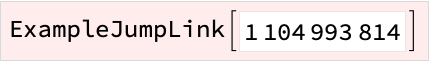0.1 horizontal space between arrows that originate from the same level ArrowStyle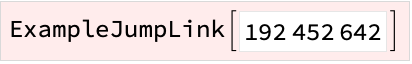{} style specifications for the arrows ExtraArrows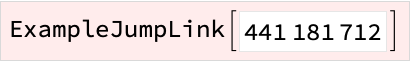{} additional arrows to be drawn that are not specified by the Hamiltonian FLabelNone whether to label the F values of the levels JLabelNone whether to label the J values of the levels LevelLength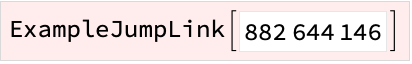0.8 length of each level LevelOffsetNone additional horizontal offsets for the levels LevelSpacing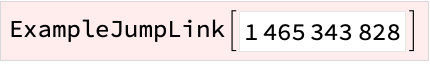0.2 horizontal space between Zeeman sublevels LevelStyle{} style specifications for the levels MaxArcSlope0.2 maximum slope below which arrows are drawn with a curve MinLabelSpacing0.3 minimum vertical spacing between the level labels MLabel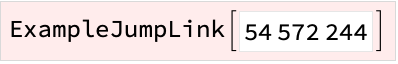None type of labels for the M values of the levels MLabelPosition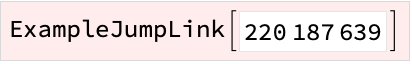Automatic where to put the M labels. ParityOffset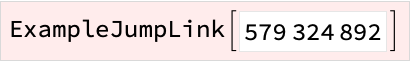Automatic whether to offset Even and Odd parity levels horizontally PopulationFunction Automatic function used to draw the populations PopulationStyle {} style specifications for populations QuantumNumberSubscripts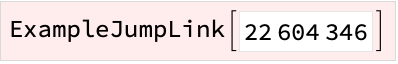{True, True, False} whether to label quantum number symbols with the StateLabel QuantumNumberSymbols{J, F, M} symbols to use for the J, F, and M quantum number labels SuppressOffResonantCouplingsTrue whether to drop couplings that would be suppressed in the rotating-wave approximation

# Examples

open allclose all

## Basic Examples(4)

A level diagram using default values for level energies:

Energies specified using the Energy parameter of AtomicState:

Energies and couplings specified using a Hamiltonian:

Specify level populations with a density matrix corresponding to an unpolarized ground state and an unpopulated upper state:

## Scope(1)

A toy system:

A Zeeman system:

A hyperfine-Zeeman system:

## Generalizations & Extensions(2)

Specify level energies with a list rather than a Hamiltonian:

Use the default numerical values for the list of energies (this gives the same behavior as when no second argument is supplied to LevelDiagram):

Specify level populations with a list rather than a density matrix:

Use lists to specify both level energies and populations:

Draw a level diagram displaying the level shifts and couplings for a magnetic-field Hamiltonian in blue, and the couplings for a electric-field Hamiltonian in red:

## Options(23)

Set the arc radius for nearly horizontal arrows:

Draw nearly horizontal arrows as straight lines by setting the arc radius to zero:

### ArrowFunction(1)

Define asystem, the Hamiltonian for interaction with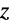-polarized light, and a Hamiltonian that displays all of the couplings for allowed spontaneous decays:

Draw the level diagram using squiggle arrows to show the allowed spontaneous decays:

### ArrowSpacing(1)

Make arrows meet at a point by setting ArrowSpacing to zero:

Space the arrows more widely:

### ArrowStyle(1)

Make dashed, purple arrows: